## 4.1.1 Topological Spaces

Recall the concepts of open and closed intervals in the set of real numbers. The open interval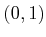includes all real numbers between 0 and, except 0 and. However, for either endpoint, an infinite sequence may be defined that converges to it. For example, the sequence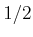,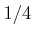,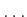,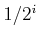converges to 0 astends to infinity. This means that we can choose a point inwithin any small, positive distance from 0 or, but we cannot pick one exactly on the boundary of the interval. For a closed interval, such as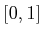, the boundary points are included.

The notion of an open set lies at the heart of topology. The open set definition that will appear here is a substantial generalization of the concept of an open interval. The concept applies to a very general collection of subsets of some larger space. It is general enough to easily include any kind of configuration space that may be encountered in planning.

A setis called a topological space if there is a collection of subsets ofcalled open sets for which the following axioms hold:

1. The union of any number of open sets is an open set.
2. The intersection of a finite number of open sets is an open set.
3. Bothand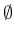are open sets.
Note that in the first axiom, the union of an infinite number of open sets may be taken, and the result must remain an open set. Intersecting an infinite number of open sets, however, does not necessarily lead to an open set.

For the special case of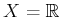, the open sets include open intervals, as expected. Many sets that are not intervals are open sets because taking unions and intersections of open intervals yields other open sets. For example, the set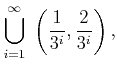(4.1)

which is an infinite union of pairwise-disjoint intervals, is an open set.

Subsections
Steven M LaValle 2012-04-20